# Shift Registers

## Digital Circuits

• #### Question 1

 Don’t just sit there! Build something!!

Learning to analyze digital circuits requires much study and practice. Typically, students practice by working through lots of sample problems and checking their answers against those provided by the textbook or the instructor. While this is good, there is a much better way.

You will learn much more by actually building and analyzing real circuits, letting your test equipment provide the “answers” instead of a book or another person. For successful circuit-building exercises, follow these steps:

1. Draw the schematic diagram for the digital circuit to be analyzed.
2. Carefully build this circuit on a breadboard or other convenient medium.
3. Check the accuracy of the circuit’s construction, following each wire to each connection point, and verifying these elements one-by-one on the diagram.
4. Analyze the circuit, determining all output logic states for given input conditions.
5. Carefully measure those logic states, to verify the accuracy of your analysis.
6. If there are any errors, carefully check your circuit’s construction against the diagram, then carefully re-analyze the circuit and re-measure.

Always be sure that the power supply voltage levels are within specification for the logic circuits you plan to use. If TTL, the power supply must be a 5-volt regulated supply, adjusted to a value as close to 5.0 volts DC as possible.

One way you can save time and reduce the possibility of error is to begin with a very simple circuit and incrementally add components to increase its complexity after each analysis, rather than building a whole new circuit for each practice problem. Another time-saving technique is to re-use the same components in a variety of different circuit configurations. This way, you won’t have to measure any component’s value more than once.

• #### Question 2

Complete the timing diagram for this circuit, assuming all Q outputs begin in the low state: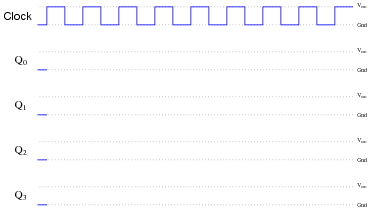• #### Question 3

Complete the timing diagram for this circuit, assuming all Q outputs begin in the low state:• #### Question 4

Complete the timing diagram for this circuit, showing propagation delays for all flip-flops (delay times much less than the width of a clock pulse), assuming all Q outputs begin in the low state: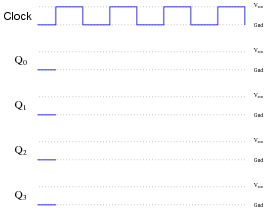• #### Question 5

What is the definition of a register in the context of digital circuitry? Also, define and compare/contrast what a shift register is.

• #### Question 6

Explain the difference between serial digital data and parallel digital data.

• #### Question 7

A helpful analogy for a shift register is a conveyor belt. Examine this illustration showing a single conveyor belt at four different times, and determine which of the following shift register operations the sequence represents:

Parallel-in, serial-out
Parallel-in, parallel-out
Serial-in, serial-out
Serial-in, parallel-out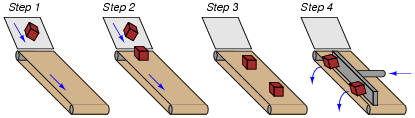• #### Question 8

A helpful analogy for a shift register is a conveyor belt. Examine this illustration showing a single conveyor belt at four different times, and determine which of the following shift register operations the sequence represents:

Parallel-in, serial-out
Parallel-in, parallel-out
Serial-in, serial-out
Serial-in, parallel-out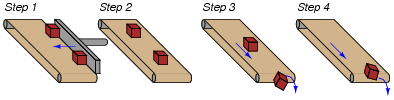• #### Question 9

A helpful analogy for a shift register is a conveyor belt. Examine this illustration showing a single conveyor belt at four different times, and determine which of the following shift register operations the sequence represents:

Parallel-in, serial-out
Parallel-in, parallel-out
Serial-in, serial-out
Serial-in, parallel-out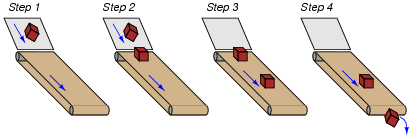• #### Question 10

Draw the schematic diagram for a five-bit serial-in/serial-out shift register circuit, and be prepared to give a brief explanation of how it functions.

• #### Question 11

Draw the necessary connecting wires between flip-flops so that serial data is shifted from right to left instead of left to right as you may be accustomed to seeing in a shift register schematic:Be sure to also note where data enters this shift register, and where data exits.

• #### Question 12

Explain how a shift register circuit could be built from D-type flip-flops with the ability to shift data either to the right or to the left, on command. I’m not necessarily asking for a schematic diagram so much as I’m looking for an explanation of how such a circuit might be built. Of course, if your best way of presenting your idea is to draw a schematic diagram, go ahead!

• #### Question 13

Explain what a universal shift register is. The 74194 is an example of a TTL universal shift register, so you will find that datasheet very helpful in answering this question.

• #### Question 14

An important function in computer circuitry is serial-to-parallel data conversion, where a stream of serial data is “read” one bit at a time, then all bits output at once in parallel form. A shift register circuit is ideal for this application. Shown here is an eight-bit shift register circuit:Draw any necessary wires and labels showing where serial data would enter the circuit, and where parallel data would exit.

• #### Question 15

Suppose we wished to use a shift register circuit to input several binary bits at once (parallel data transfer), and then output the bits one at a time over a single line (serial data transfer). You should be aware of how shift registers are constructed with D-type flip-flops. Now, describe how we can get parallel data entered into a shift register circuit. Note: there is more than one answer to this question!

• #### Question 16

A binary number is parallel-loaded into a shift register. The shift register is then commanded to “shift right” for one clock pulse. How does the value of the shifted binary number compare to the number originally loaded in, assuming that the MSB is on the very left flip-flop of the shift register?

• #### Question 17

Switch contact bounce is often a problem when connecting mechanical contact switches or relays to the inputs of digital semiconductor circuits. When a switch transitions from open to closed, or from closed to open, there is usually a burst of on/off pulses rather than a single, crisp, change of logic state: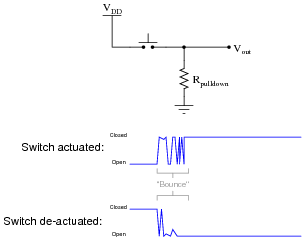Digital electronic circuits, of course, react to these pulses as though they were very rapid actuations/de-actuations of the switch. This may cause problems, especially in applications where a mechanical switch input causes a counter to increment or decrement!

To fix this problem we must properly condition the switch signal to eliminate the spurious on/off pulses. The process of doing this is called debouncing. There is more than one way to de-bounce a switch, but one of the more sophisticated ways uses a serial-in, serial-out shift register with an asynchronous reset (clear) input:Explain how this circuit works to de-bounce the switch’s “dirty” signal, producing a “clean” (de-bounced) signal for a subsequent digital circuit’s input.

• #### Question 18

A mechanically inclined friend of yours wishes to build an automated water fountain, where ten water jets are turned on in sequence, one at a time. Each water jet is controlled by a solenoid valve, energized by 120 volt AC line power.

Your friend understands how to wire up the solenoid valves and build all the plumbing to make the fountain work. He also understands how to interpose power to the solenoid valve coils using small relays, so a digital control circuit operating at a low DC supply voltage will be able to energize the valves. The only problem is, this friend of yours does not know how to build a circuit to do the sequencing. How do you turn on one out of ten outputs at a time, in sequence?

Another friend who is studying digital circuits has a solution to the design problem. She says you could generate a one-out-ten sequence by using a BCD counter (4 bit) driving a 4-line to 16-line decoder. A simple 555 astable multivibrator circuit could supply the necessary clock pulses, and the decoder outputs could drive the relays, and then the solenoid valves, as fast as you wanted.

However, your instructor just recently told you about a different way to generate a one-out-of-n counting sequence by using shift registers: the circuit is called a ring counter. A ring counter would use fewer parts than the counter/decoder idea. Explain what a ring counter is, and how it would work in this application.

• #### Question 19

An analog-to-digital converter is a circuit that inputs an analog signal and outputs a multiple-bit binary number equivalent to that signal’s amplitude:A free-running analog-to-digital converter is one that updates its digital output as often as it can, not waiting for any prompting from another device. If we were to connect a free-running ADC to a computer (microprocessor or microcontroller), we would need some way to sample the ADC’s output at times specified by the computer, and hold that binary number long enough for the computer to register it. Otherwise, the ADC may update its output in the middle of one of the computer’s “input” cycles, possibly resulting in corrupted data.

We could build such a sample-and-hold circuit out of flip-flops. What type of flip-flop would we use for this purpose, and how many would we need for the ADC circuit shown above? This circuit we would build is also known as a shift register. What kind of shift register inputs multiple bits of data all at once, and transfers that data to its output lines all at once, at the command of a clock pulse?

• #### Question 20

This shift register circuit energizes one LED at a time (beginning with the bottom LED at power-up), in a rotating pattern that moves at the pace of the clock:A technician decides to reverse the direction of pattern motion, and alters the circuit as such:Unfortunately, this does not work as planned. Now, the bottom LED blinks once upon power-up, then all LEDs remain off. What did the technician do that was incorrect? What needs to be done to fix the problem?

• #### Question 21

This shift register circuit produces a sequential light pattern reminiscent of the old Mercury Cougar tail-lights: first one LED energizes, then two LEDs energize, and then all three LEDs energize before all de-energizing and repeating the sequence. The 74HC194 shift register circuit is set to always operate in the “shift right” mode with the shift-right serial input (DSR) tied high, the master reset ([MR]) input used to set all output lines to a low state at the end of each cycle:The sequential light pattern is supposed to begin whenever the “Trigger” input momentarily goes high. Unfortunately, something has failed in this circuit which is preventing any of the LEDs to come on. No blinking light sequence ensues, no matter what the state of the “Trigger” input.

Identify some likely failures in this circuit that could cause this to happen, other than a lack of power supply voltage. Explain why each of your proposed faults would cause the problem, and also identify how you would isolate each fault using test equipment.

• #### Question 22

This shift register circuit drives the four coils of a unipolar stepper motor, one at a time, in a rotating pattern that moves at the pace of the clock. The drive transistor circuitry (Q1, Q2, and resistors R2 through R6) are shown only for one of the four coils. The other three shift register outputs have identical drive circuits connected to the respective motor coils:Suppose this stepper motor circuit worked just fine for several years, then suddenly stopped working. Explain where you would take your first few measurements to isolate the problem, and why you would measure there.